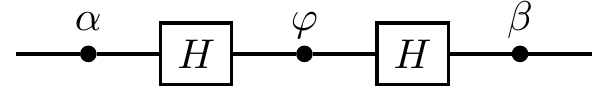## 2.8 Any unitary operation on a single qubit

There are infinitely many single-qubit unitaries, i.e. unitary operations that can be performed on a single qubit. In general, any complex (n\times n) matrix has n^2 complex entries, and can thus be specified by 2n^2 real independent parameters.50 The unitarity constraint removes n^2 of these (why? the argument is that once we specify n^2 parameters, the rest are uniquely determined by solving the equation that needs to be satisfied in order for the matrix to be unitary). So any unitary (n\times n) matrix has n^2 real independent parameters.

This sort of argument — counting how many parameters determine a family of matrices — is really an example of calculating the dimension of a vector space. More generally, saying things like “imposing a polynomial equation condition on the coefficients lowers the number of (complex) parameters necessary by 1” is the bread and butter of algebraic geometry, where we try to understand how satisfying polynomial equations can be interpreted as geometrically modifying high-dimensional “shapes”.

In particular, we need four real parameters to specify a (2\times 2) unitary matrix. If we are prepared to ignore global phase factors (which we are!) then there are only three real parameters left. The real question is, can we use this to construct and implement any unitary on a single qubit in some simple way?

Delightfully, the answer is yes, we can.

Any unitary operation on a qubit (up to an overall multiplicative phase factor) can be implemented by a circuit containing just two Hadamards and three phase gates, with adjustable phase settings, as in Figure 2.3.Figure 2.3: The universal circuit for unitary (2\times2) matrices, exhibiting how any such matrix is uniquely determined (up to a global phase) by three real parameters.

If we multiply the matrices51 corresponding to each gate in the network we obtain the single matrix U(\alpha,\beta,\varphi) =\begin{bmatrix} e^{-i\left(\frac{\alpha+\beta}{2}\right)}\cos\varphi/2 & -ie^{i\left(\frac{\alpha-\beta}{2}\right)}\sin\varphi/2 \\-ie^{-i\left(\frac{\alpha-\beta}{2}\right)}\sin\varphi/2 & e^{i\left(\frac{\alpha+\beta}{2}\right)}\cos\varphi/2 \end{bmatrix}. Any (2\times 2) unitary matrix (up to global phase) can be expressed in this form using the three independent real parameters, \alpha, \beta, and \varphi, which take values in [0,2\pi]. In order to see that this construction does what it claims, let us explore an intriguing mathematical connection between single-qubit unitaries and rotations in three dimensions.

1. Any complex number z is uniquely specified by two real parameters, writing z=x+iy or z=re^{i\varphi}, for example. This is an instance of the fact that \mathbb{C} is a two-dimensional vector space over \mathbb{R}.↩︎

2. Remember that the order of matrix multiplication is reversed when compared to reading circuit diagrams.↩︎